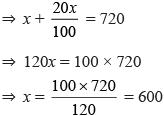Courses

Olympiad Test: Mathematical Reasoning - 2

10 Questions MCQ Test Mathematics Olympiad Class 7 | Olympiad Test: Mathematical Reasoning - 2

Description
Attempt Olympiad Test: Mathematical Reasoning - 2 | 10 questions in 20 minutes | Mock test for Class 7 preparation | Free important questions MCQ to study Mathematics Olympiad Class 7 for Class 7 Exam | Download free PDF with solutions
QUESTION: 1

By selling an item in Rs. 720, Manish gains 20%. What is its cost price?

Solution:

Let x be the cost price.
⇒ x + 20% of x = 720QUESTION: 2

Manoj is twice as old as Monu. 3 years ago Manoj was three times as old as Monu. What is the present age of Manoj?

Solution:

Let Monu’s age = x
Manoj’s age = 2x
A/Q,  2x – 3 = 3 (x – 3)
⇒ 2x – 3 = 3x – 9
⇒ 3x – 2x = 9 – 3
⇒ x = 6
∴ Present age of Manoj = 2 × 6 = 12 years.

QUESTION: 3

What is the product of all the numbers in the dial of a telephone?

Solution:

The product of all the numbers in the dial of a telephone = 0

QUESTION: 4

At the end of a meeting the ten persons present, all shake hands with each other once. What is the total number of handshakes altogether?

Solution:

Total number of handshakes
= 9 + 8 + 7 + 6 + 5 + 4 + 3 + 2 + 1 = 45

QUESTION: 5

Direction: If + means ×, × means –, ÷ means + and ‘–‘ means ÷, then find the value in each of the following

175 – 25 ÷ 5 + 20 × 3 + 10 = ?

Solution:

175 – 25 ÷ 5 + 20 × 3 + 10
= 175 ÷ 25 + 5 × 20 – 3 × 10
= 7 + 5 × 20 – 3 × 10
= 7 + 100 – 30
= 107 – 30 = 77

QUESTION: 6

Direction: If + means ×, × means –, ÷ means + and ‘–‘ means ÷, then find the value in each of the following

225 – 15 + 9 × 15 ÷ 3 = ?

Solution:

225 – 15 + 9 × 15 ÷ 3
= 225 ÷ 15 × 9 – 15 + 3
= 15 × 9 – 15 + 3
= 135 – 15 + 3 = 123

QUESTION: 7

Direction: If + means ×, × means –, ÷ means + and ‘–‘ means ÷, then find the value in each of the following

23 ÷ 107 × 135 – 5 + 3 = ?

Solution:

23 ÷ 107 × 135 – 5 + 3
= 23 + 107 – 135 ÷ 5 × 3
= 23 + 107 – 27 × 3
= 23 + 107 – 81
= 130 – 81 = 49

QUESTION: 8

Direction: If + means ×, × means –, ÷ means + and ‘–‘ means ÷, then find the value in each of the following

297 × 57 × 345 – 15 + 11 ÷ 18

Solution:

297 × 57 × 345 – 15 + 11 ÷ 18
= 297 – 57 – 345 ÷ 15 × 11 + 18
= 297 – 57 – 23 × 11 + 18
= 297 – 57 – 253 + 18
= 315 – 310 = 5

QUESTION: 9

Direction: If + means ×, × means –, ÷ means + and ‘–‘ means ÷, then find the value in each of the following

78 – 13 + 7 × 6 ÷ 17 + 3

Solution:

78 – 13 + 7 × 6 ÷ 17 + 3
= 78 ÷ 13 × 7 – 6 + 17 × 3
= 6 × 7 – 6 + 17 × 3
= 42 – 6 + 51
= 93 – 6 = 87

QUESTION: 10

Direction: If + means ×, × means –, ÷ means + and ‘–‘ means ÷, then find the value in each of the following

123 × 4 + 7 ÷ 76 – 19 + 7

Solution:

123 × 4 + 7 ÷ 76 – 19 + 7
= 123 – 4 × 7 + 76 ÷ 19 × 7
= 123 – 4 × 7 + 4 × 7
= 123 – 28 + 28
= 123Use Code STAYHOME200 and get INR 200 additional OFF Use Coupon Code

Track your progress, build streaks, highlight & save important lessons and more!

Similar ContentRelated tests